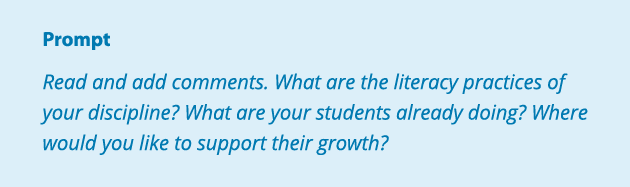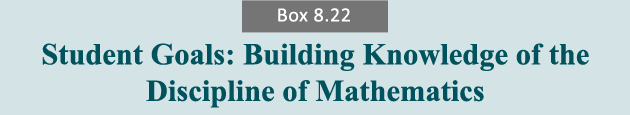Full Summaries Sorted

# Reading Apprenticeship Goals in MathematicsIn a mathematics classroom, students learn about the discipline of mathematics and themselves as readers and users of mathematics by way of the following discipline-speciﬁc goals. CONCEPTUAL CATEGORIES* I can identify the purpose for and use diﬀerent areas of math knowledge such as number, algebra, functions, geometry, statistics and probability, and modeling. MATHEMATICAL REASONING I can think interchangeably about a math problem in abstract and quantitative terms. I monitor the reasonableness of the relationship between my abstract and quantitative thinking. MATHEMATICAL REPRESENTATION I can read and represent mathematics with words, formulas, and mathematical symbols. I can read and create diagrams, tables, graphs, and ﬂowcharts for mathematical purposes. MATHEMATICAL LANGUAGE I understand the precise nature of mathematical language and use it to communicate exactly. PROBLEM IDENTIFICATION I can read and identify “the problem” in a math problem. PROBLEM SOLVING I make conjectures about and evaluate alternative approaches to a problem and then monitor the reasonableness of a solution approach as it proceeds. ACCURACY I understand that in mathematics there may be alternate approaches to a solution, but only one correct answer. I check that the ﬁnal solution makes sense and all computation is correct. PATTERN APPLICATION I look for mathematical structures, approaches, and patterns that I can apply to the solution of new problems. MATHEMATICAL IDENTITY I am aware of my evolving identity as a reader and user of mathematics. *These conceptual categories are drawn from the Common Core State Standards for Mathematical Practice.
 From Reading for Understanding, pp 282-284

DMU Timestamp: February 06, 2019 23:03

Image
change display
Video
• Clickicons on the left to see existing comments.
Logging in, please wait...